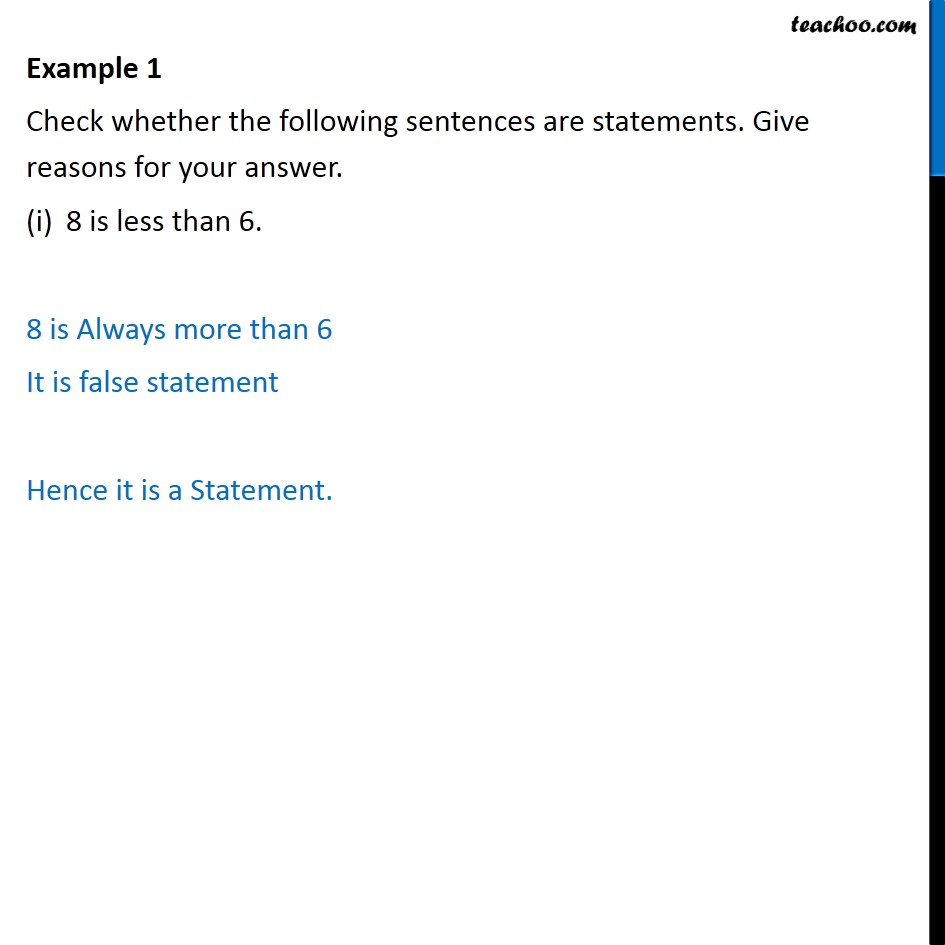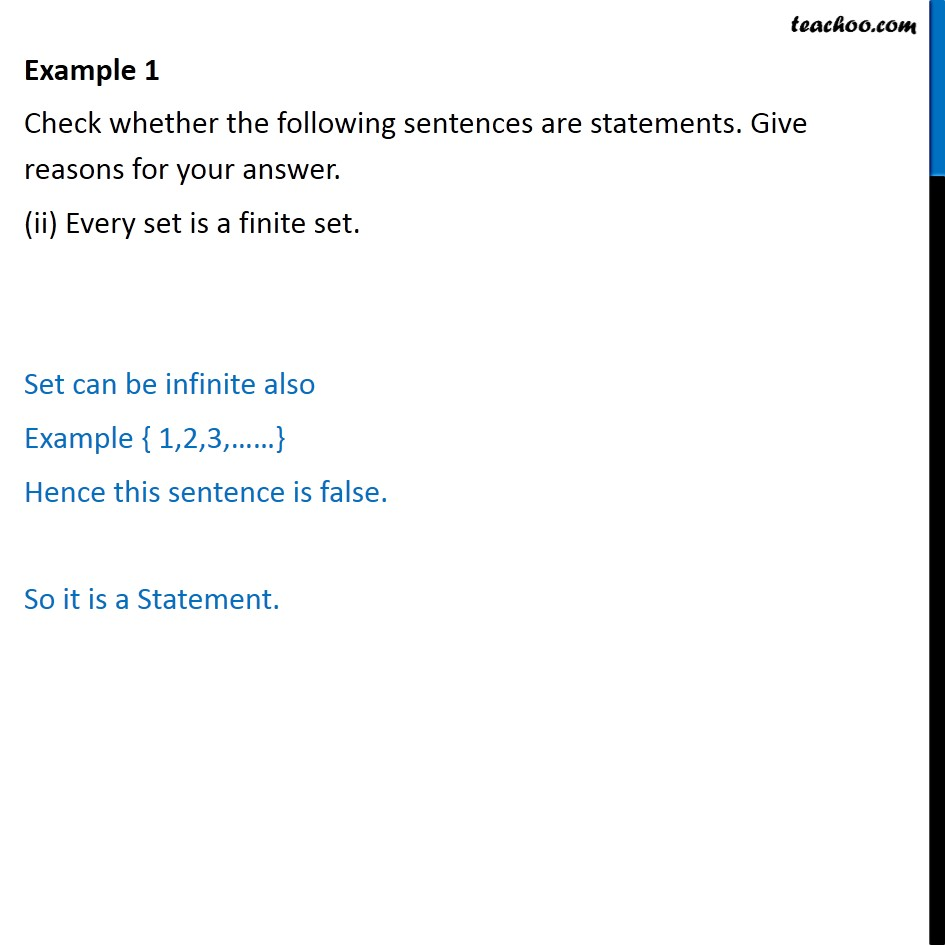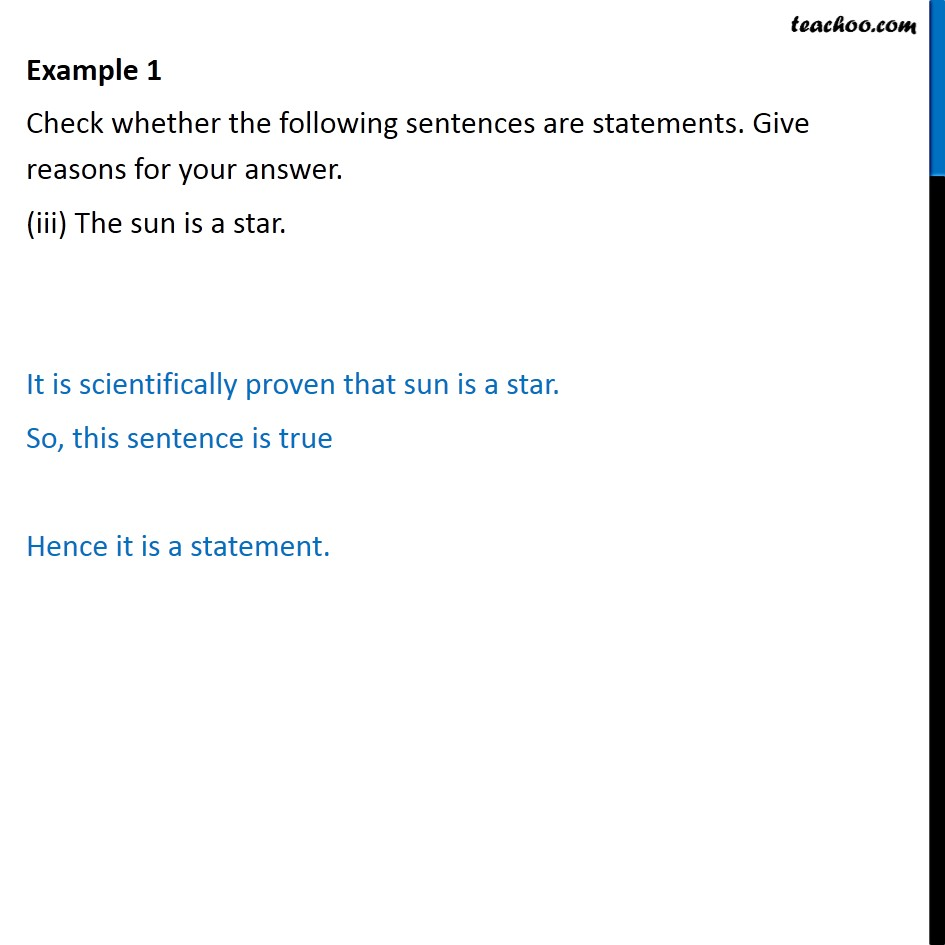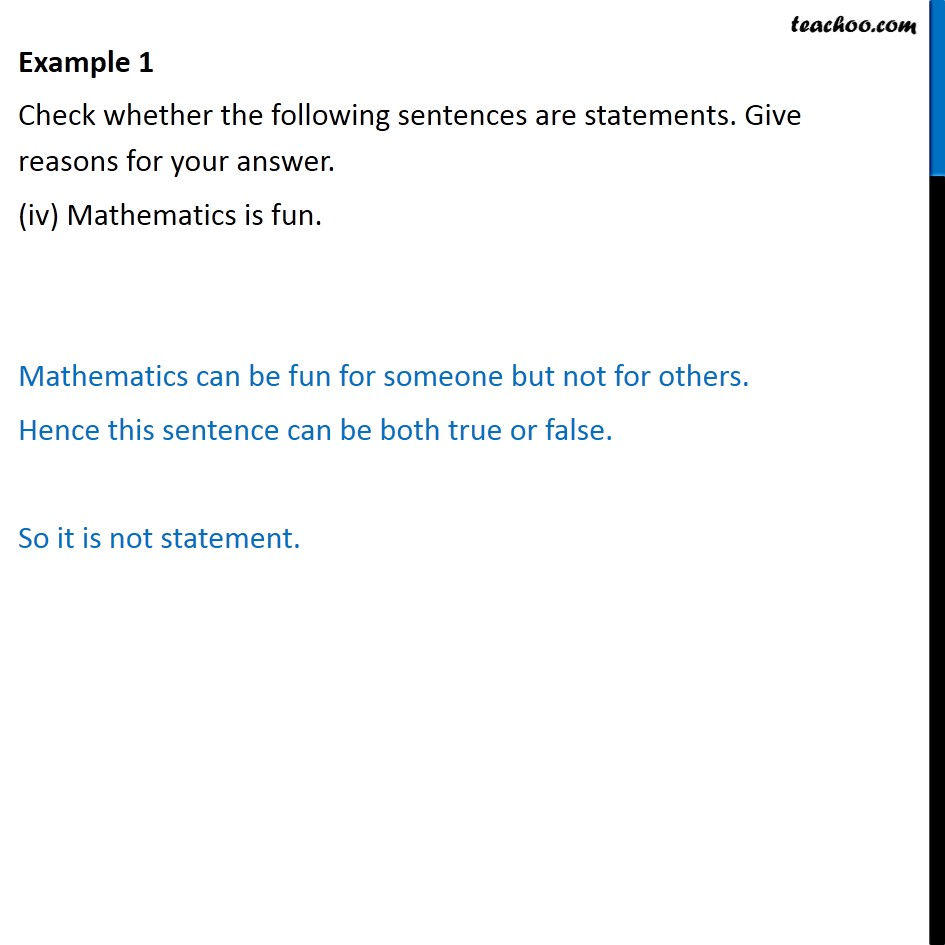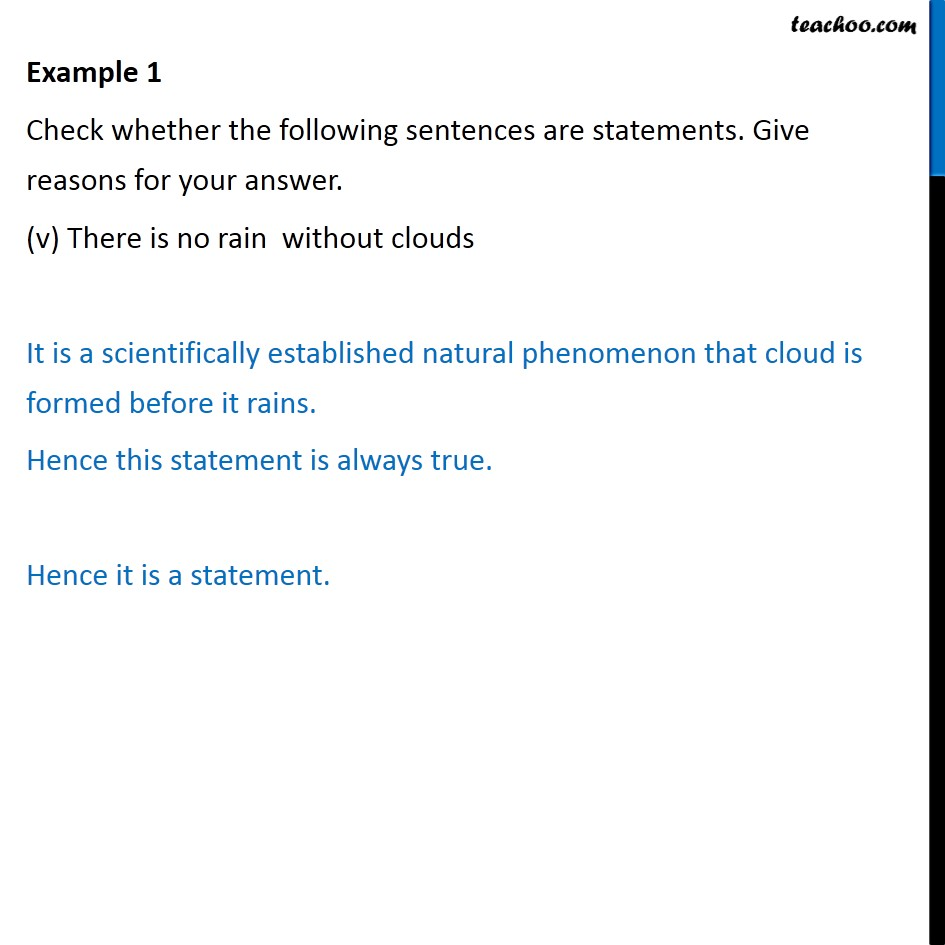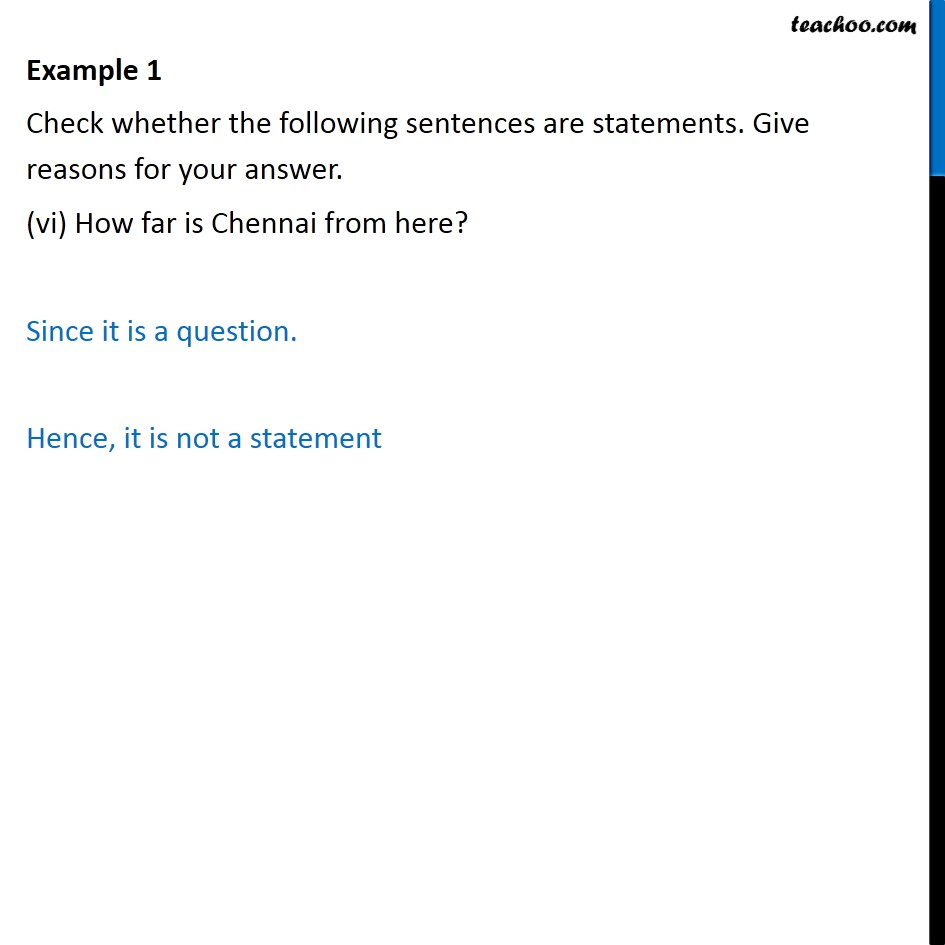1. Chapter 14 Class 11 Mathematical Reasoning
2. Concept wise
3. Statements

Transcript

Example 1 Check whether the following sentences are statements. Give reasons for your answer. 8 is less than 6. 8 is Always more than 6 It is false statement Hence it is a Statement. Example 1 Check whether the following sentences are statements. Give reasons for your answer. (ii) Every set is a finite set. Set can be infinite also Example { 1,2,3, } Hence this sentence is false. So it is a Statement. Example 1 Check whether the following sentences are statements. Give reasons for your answer. (iii) The sun is a star. It is scientifically proven that sun is a star. So, this sentence is true Hence it is a statement. Example 1 Check whether the following sentences are statements. Give reasons for your answer. (iv) Mathematics is fun. Mathematics can be fun for someone but not for others. Hence this sentence can be both true or false. So it is not statement. Example 1 Check whether the following sentences are statements. Give reasons for your answer. (v) There is no rain without clouds It is a scientifically established natural phenomenon that cloud is formed before it rains. Hence this statement is always true. Hence it is a statement. Example 1 Check whether the following sentences are statements. Give reasons for your answer. (vi) How far is Chennai from here? Since it is a question. Hence, it is not a statement

Statements

About the AuthorDavneet Singh
Davneet Singh is a graduate from Indian Institute of Technology, Kanpur. He has been teaching from the past 9 years. He provides courses for Maths and Science at Teachoo.